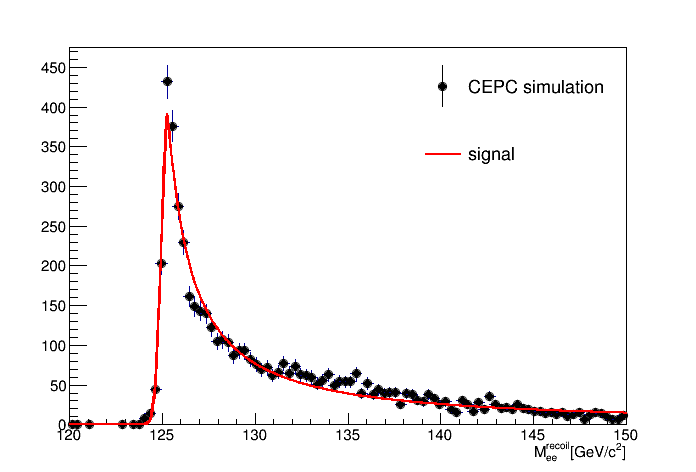# Recoil Mass of Leptons from Z

• You may find the project at http://cepcgit.ihep.ac.cn/lihawl/llH_Rec. The website is open to register. When you can access the project, use git clone git@cepcgit.ihep.ac.cn:lihawl/llH_Rec.git to have a local copy of this project.

## Introduction

The final states of $llH$ mainly come from the $ZH$ (Higgs-strahlung), where $l$ means charged leptons, as shown in the below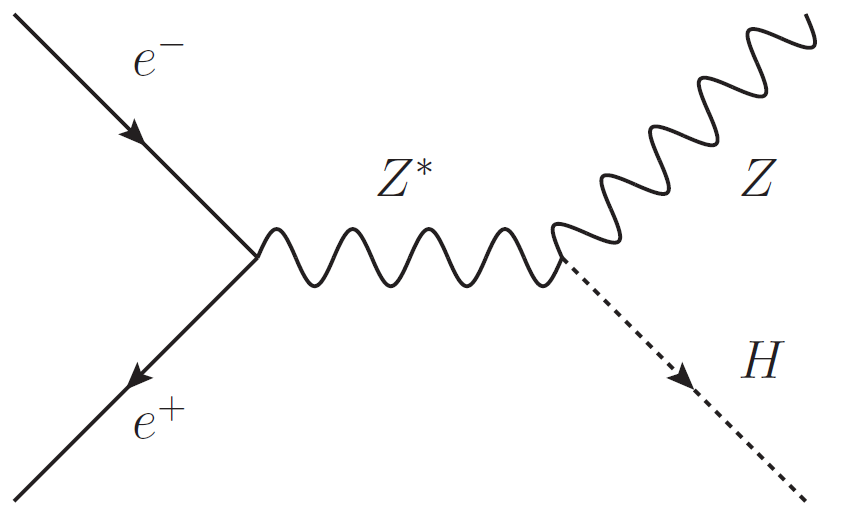The $Z$ boson can be constructed by the invariant mass of di-lepton, and the Higgs can be constructed by the recoil mass of the $Z$. This method of construction of Higgs is independent to how Higgs decays, and allows us measure the $ZH$ cross-section model indepently. You can find more details at Cross section and Higgs mass measurement with Higgsstrahlung at the CEPC.

## Event Selection

The lepton candidates are selected by the criterion

Then di-lepton candidates are selected by the criterion

If there are multiple combinations, the combination with smallest $\vert M_{ll} - M_Z\vert$ is selected. Once the di-lepton is constructed, the recoil mass is calculated by

## The Plots

The invariant mass of di-muon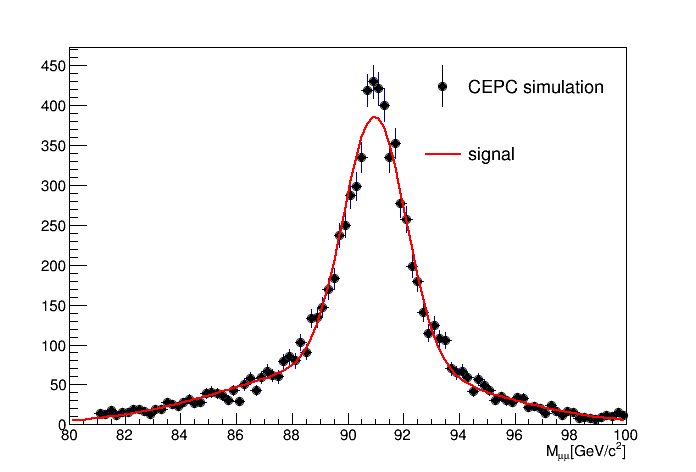The recoil mass of di-muon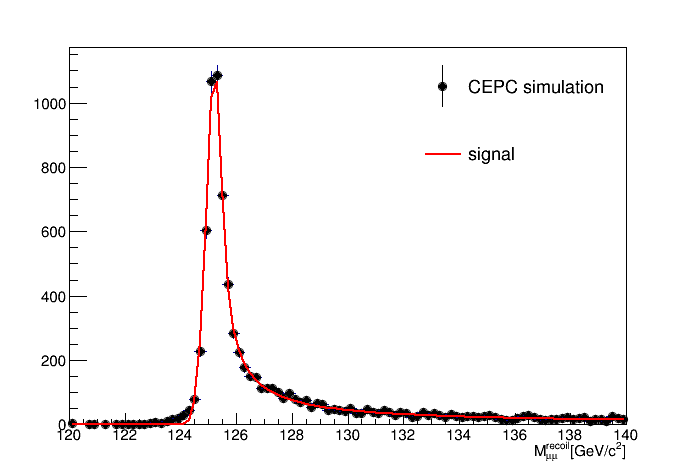The invariant mass of di-electron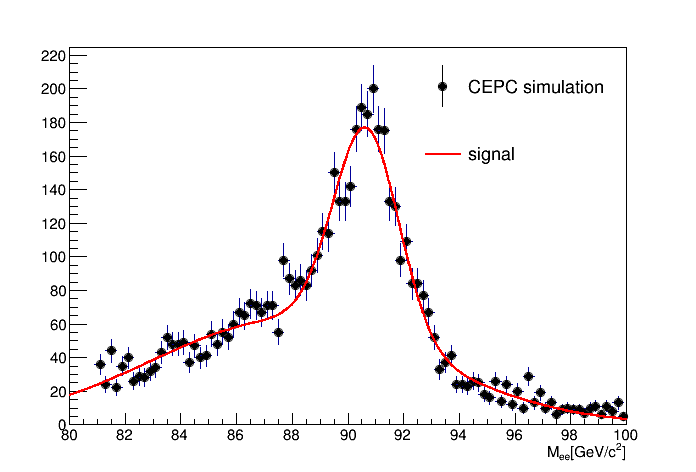The recoil mass of di-electron